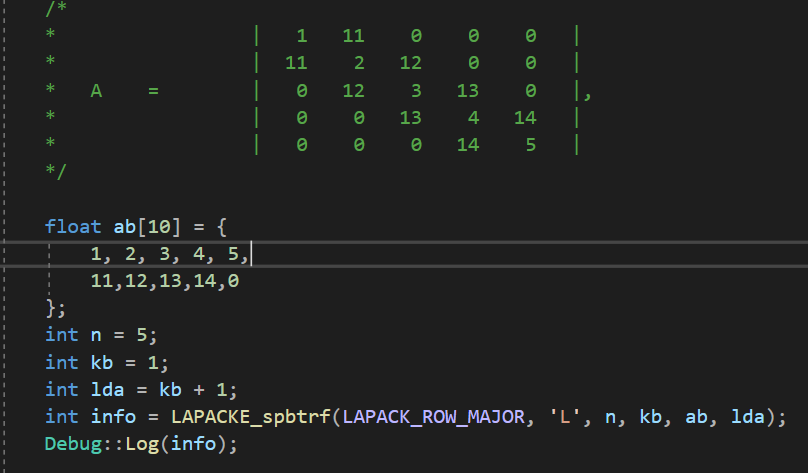Community
cancel
Showing results for
Did you mean:Novice
298 Views

## Why the "LAPACKE_spbtrf" always return "-6"?

Hi, guys, I use "LAPACKE_spbtrf" to factorize a matrix A, as the following code, but the function always return -6, which indicate that parameter i is an illegeal value(Does it mean that the "lda" is illegal?).

So why it returns -6?1 SolutionBlack Belt
280 Views

The matrix that you chose is not positive definite. The value of ldA is not correct for the 2-D array conventions that you are using. I think that you should spend time reading the MKL documentation. The MKL distribution comes with a number of example codes that are ready to compile, link and run.

Here is C code that worked (I changed the matrix to make it +def.).

``````#include <stdio.h>
#include <mkl.h>

int main() {

float ab = { 30,31,32,33,34,
11,12,13,14,0 };
int i,j;
const int n = 5;
int kd = 1;
int lda = n;
int info = LAPACKE_spbtrf(LAPACK_ROW_MAJOR, 'L', n, kd, ab, lda);
printf("info = %d\n",info);
for(j=0; j<=kd; j++){
for(i=0; i<n; i++)printf("%8.5f ",ab[n*j+i]);
printf("\n");
}
return 0;
}
``````

With such small examples, it is often instructive to use a package such as Matlab so that you can compare solutions, and to construct suitable input matrices. In this case, I increased the values on the principal diagonal, starting with your values, until Matlab told me that the matrix was positive definite.

2 RepliesBlack Belt
281 Views

The matrix that you chose is not positive definite. The value of ldA is not correct for the 2-D array conventions that you are using. I think that you should spend time reading the MKL documentation. The MKL distribution comes with a number of example codes that are ready to compile, link and run.

Here is C code that worked (I changed the matrix to make it +def.).

``````#include <stdio.h>
#include <mkl.h>

int main() {

float ab = { 30,31,32,33,34,
11,12,13,14,0 };
int i,j;
const int n = 5;
int kd = 1;
int lda = n;
int info = LAPACKE_spbtrf(LAPACK_ROW_MAJOR, 'L', n, kd, ab, lda);
printf("info = %d\n",info);
for(j=0; j<=kd; j++){
for(i=0; i<n; i++)printf("%8.5f ",ab[n*j+i]);
printf("\n");
}
return 0;
}
``````

With such small examples, it is often instructive to use a package such as Matlab so that you can compare solutions, and to construct suitable input matrices. In this case, I increased the values on the principal diagonal, starting with your values, until Matlab told me that the matrix was positive definite.Novice
263 Views

Hi, Mecej, thanks very much again!

I didn't understand the "lda" well before, that's the main problem. Also a wrong intuition about the format that a positive definite band matrix have.

Now all the program works well! Thanks!